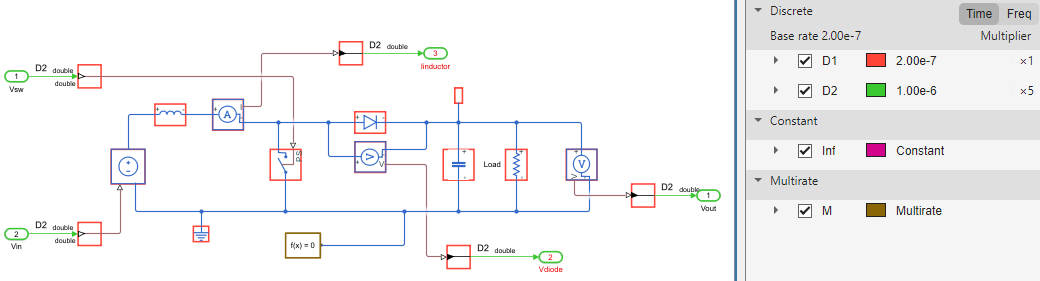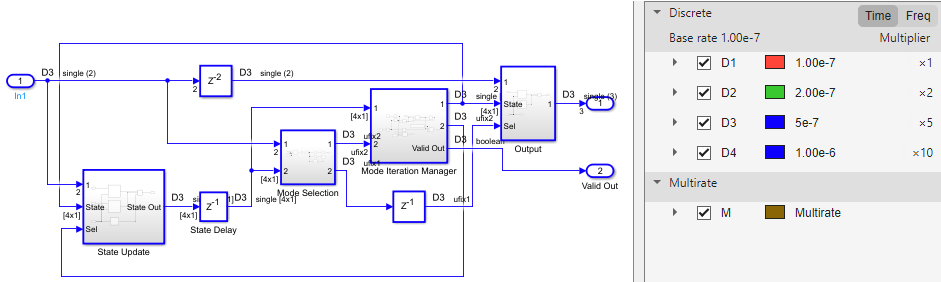## Improve Sampling Rate of HDL Implementation Model Generated from Simscape Algorithm

If you design your algorithm by using Simscape™ switched linear blocks, you can run the Simscape HDL Workflow Advisor to generate an HDL implementation model. When you open the HDL implementation model, you see the HDL algorithm that models the state-space representation by using Simulink® blocks that are compatible for HDL code generation. To learn more about the Simscape HDL Workflow Advisor, see Simscape HDL Workflow Advisor Tasks.

### Sampling Frequency

When you generate HDL code and deploy the plant model onto an FPGA, you may want to improve the sampling frequency. The sampling frequency depends on these parameters:

• FPGA clock frequency

• Oversampling factor

• Number of solver iterationsTo improve the sampling rate, you want to maximize the FPGA clock frequency, and minimize the oversampling factor and number of solver iterations. As you improve the sampling rate, make sure that the updated sampling frequency is equivalent to the fixed sample time that you specify for your original Simscape model by using the Solver Configuration block. To learn more about how this block is used in your model before running the Simscape HDL Workflow Advisor, see Generate HDL Code for Simscape Models.

The preceding section uses the boost converter model as an example to illustrate how you can modify the oversampling factor and the number of solver iterations to improve the sampling rate.

### Boost Converter Model

This example uses the boost converter model to illustrate the change in sample time in the generated HDL implementation model and the oversampling factor that is saved on the model.

1. Open the boost converter model. To learn how the boost converter is implemented, open the `Simscape_system` Subsystem. To open the boost converter model, in the MATLAB® Command Window, enter:

```open_system('sschdlexBoostConverterExample') open_system('sschdlexBoostConverterExample/Simscape_system')```You see that the model runs at a sample time `1e-6`. The sample time of `200e-9` corresponds to the sample time of the sources that drive the Simscape algorithm.

`sschdladvisor('sschdlexBoostConverterExample')`
3. Run the workflow to the Generate implementation model task.

After running this task, you see a link to the generated HDL implementation model. Click the link to open the HDL implementation model.

4. Simulate the HDL implementation model. When you navigate the model to the `HDL Algorithm` Subsystem, you see that the model uses `single` data types and runs at a sample time `200e-9`, which is `5` times faster than the original Simscape model.5. Run this command to see the HDL parameter settings that are saved on the model:

`hdlsaveparams('gmStateSpaceHDL_sschdlexBoostConverterExamp')`

```%% Set Model 'gmStateSpaceHDL_BoostConverter_HDL' HDL parameters hdlset_param('gmStateSpaceHDL_BoostConverter_HDL', 'FloatingPointTargetConfiguration', ... hdlcoder.createFloatingPointTargetConfig('NativeFloatingPoint' ... , 'LatencyStrategy', 'MIN') ... ); hdlset_param('gmStateSpaceHDL_BoostConverter_HDL', 'HDLSubsystem', ... 'gmStateSpaceHDL_BoostConverter_HDL'); hdlset_param('gmStateSpaceHDL_BoostConverter_HDL', 'MaskParameterAsGeneric', 'on'); hdlset_param('gmStateSpaceHDL_BoostConverter_HDL', 'Oversampling', 60); % Set SubSystem HDL parameters hdlset_param('gmStateSpaceHDL_BoostConverter_HDL/HDL Subsystem', 'FlattenHierarchy', 'on'); % Set SubSystem HDL parameters hdlset_param('gmStateSpaceHDL_BoostConverter_HDL/HDL Subsystem/HDL Algorithm/State Update/Multiply State', ... 'SharingFactor', 1); ```

The HDL parameters that are saved indicate that the model has the native floating-point mode enabled and uses an Oversampling factor of `60` and has Latency Strategy set to `MIN`. This default values chosen for number of solver iterations and combination of HDL parameters offers an optimal trade-off between oversampling factor and the target FPGA clock frequency and improves the sampling frequency. To further improve the sampling frequency, reduce the number of iterations and the oversampling factor.

### Reducing Number of Solver Iterations

For each mode in the physical system, the switched linear workflow arrives at a state-space representation. The solver method is iterative and performs multiple computations to determine the correct mode for the next time step. After a certain number of iterations, the output value from the next time step becomes the same as the value from the previous time step. This consistency in the output value indicates the correct number of solver iterations.

The Advisor by default chooses an optimal value for the number of solver iterations. See Using Number of Solver Iterations. To improve the sampling rate, reduce the number of solver iterations. The number of solver iterations depends on various factors such as the complexity of your design, the number of modes in the design that the workflow calculates, and so on.

In the Generate implementation model task of the Simscape HDL Workflow Advisor:

1. Start by reducing the Number of solver iterations to a value such as `3`

2. Select Generate validation logic for the implementation model, and then generate the HDL implementation model.

3. Simulate the HDL implementation model and open the Diagnostic Viewer to verify that the model does not display warnings or assertions.

If you see warnings or assertions, it indicates a simulation mismatch because the number of solver iterations that you specified is not adequate to compute the required number of modes in the state-space design. Resolve the mismatch by increasing the validation logic tolerance value or the number of solver iterations. Changing Floating-point precision to `double` is not recommended. Double-precision operations have large latencies and require a large Oversampling factor to allocate sufficient delays, which reduces the sampling frequency. See Validate HDL Implementation Model to Simscape Algorithm.

### Using Oversampling Factor and Latency Strategy

The Oversampling factor specifies the factor by which the FPGA clock rate is a multiple of the HDL implementation model base sample rate. The HDL implementation model contains feedback loops and performs multiplication of large matrices that have floating-point data types inside the feedback loops. To accommodate the large latency introduced by these floating-point operations inside the feedback loops, the code generator uses a large value of oversampling factor in conjunction with the clock-rate pipelining optimization on the model. For more information, see Strategy 1: Global Oversampling.

You vary the oversampling factor and latency strategy of the floating-point operator in conjunction. The default oversampling factor of `60` and minimum latency strategy gives an optimal sampling frequency. To achieve the maximum FPGA clock frequency, use the maximum latency strategy. When you specify this latency strategy, the floating-point operations introduce the maximum number of delays. To allocate these delays, increase the oversampling factor. If the increase in FPGA clock frequency outweighs the increase in oversampling factor, you achieve a higher sampling frequency.

To change the latency strategy and oversampling factor in conjunction from the Configuration parameters dialog box:

1. On the HDL Code Generation > Floating Point pane, change the Latency Strategy to `Max` .

2. On the HDL Code Generation > Global Settings pane, increase the Oversampling factor to a value such as `100` depending on the complexity of your HDL design.

For the boost converter model, the default settings of Number of solver iterations set to `5`, Oversampling factor set to `60`, and Latency Strategy set to `Min` provides the optimal sampling frequency.